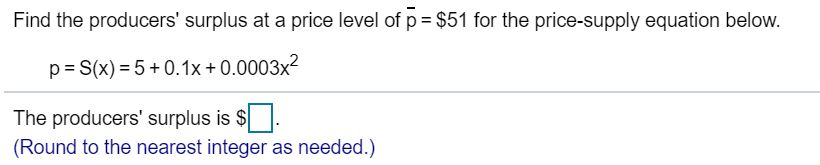# Find the producers' surplus at a price level of p \$51 for the price-supply equation below.S(x) 5+0.1x + 0.0003x2pThe producers' surplus is \$(Round to the nearest integer as needed.)

Question
87 viewshelp_outlineImage TranscriptioncloseFind the producers' surplus at a price level of p \$51 for the price-supply equation below. S(x) 5+0.1x + 0.0003x2 p The producers' surplus is \$ (Round to the nearest integer as needed.) fullscreen
check_circle

Step 1

Given price-supply equation is,

Step 2

Differentiating the price-supply equation with respect to x

Step 3

Now finding the value of x at which the ...

### Want to see the full answer?

See Solution

#### Want to see this answer and more?

Solutions are written by subject experts who are available 24/7. Questions are typically answered within 1 hour.*

See Solution
*Response times may vary by subject and question.
Tagged in

### Derivative